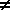Home    |    Teacher    |    Parents    |    Glossary    |    About UsEmail this page to a friendResources· Cool Tools · Formulas & Tables · References · Test Preparation · Study Tips · Wonders of MathSearchBasic Identities (Math | Algebra | Basic Identities)

Sum (or difference) of 2 real numbers equals a real number

a + 0 = a

a + (-a) = 0

(a + b) + c = a + (b + c)

a + b = b + a

Definition of Subtraction

a - b = a + (-b)Closure Property of Multiplication

Product (or quotient if denominator0) of 2 reals equals a real number

Multiplicative Identity

a * 1 = a

Multiplicative Inverse

a * (1/a) = 1     (a0)

(Multiplication times 0)

a * 0 = 0

Associative of Multiplication

(a * b) * c = a * (b * c)

Commutative of Multiplication

a * b = b * a

Distributive Law

a(b + c) = ab + ac

Definition of Division

a / b = a(1/b)